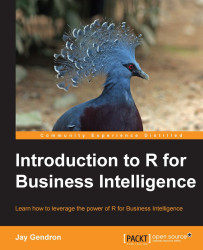•#### Introduction to R for Business Intelligence#### Overview of this book

Introduction to R for Business IntelligenceCreditsAcknowledgementwww.PacktPub.comPrefaceFree ChapterData CleaningExploratory Data AnalysisData Mining with Cluster AnalysisTime Series AnalysisVisualizing the Datas StoryWeb Dashboards with ShinyReferencesR Packages Used in the BookR Code for Supporting Market Segment Business Case Calculations## Using a simple linear regression

You built a linear regression model by simply using the `lm()` function on your data. You also used the LINE approach to make sure that your model satisfied the assumptions of linear regression. Building SLRs is often straightforward, but it is very important that you know how to interpret the output.

### Interpreting model output

There is a lot of information available within a linear regression model. You can see an expanded output by using the `summary()` function:

```summary(model1)
```

The following is the output:

```Call:
lm(formula = revenues ~ marketing_total, data = adverts)
Residuals:
Min      1Q  Median      3Q     Max
-8.6197 -1.8963 -0.0006  2.1705  9.3689
Coefficients:
Estimate Std. Error t value Pr(>|t|)
(Intercept)     32.006696   0.635590   50.36   <2e-16 ***
marketing_total  0.051929   0.002437   21.31   <2e-16 ***
---
Signif. codes:  0 '***' 0.001 '**' 0.01 '*' 0.05 '.' 0.1 ' ' 1
Residual standard error: 3.054 on 170 degrees...```